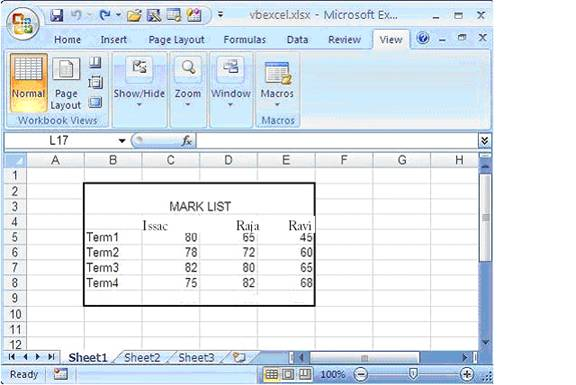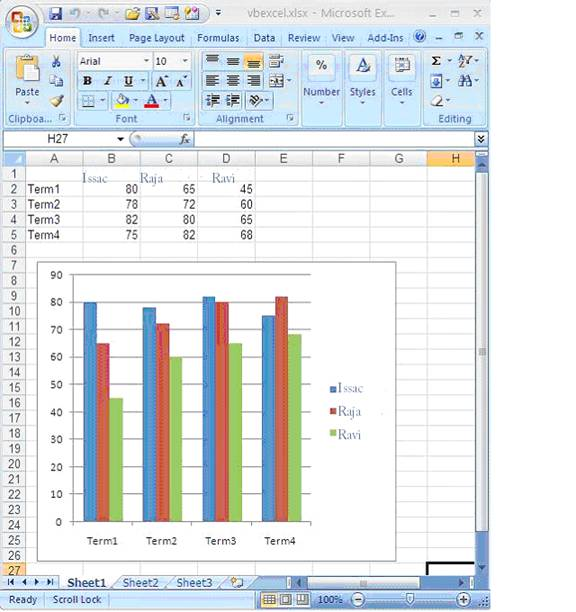# Programming Tutorials

## Using Excel 2007 Chart in VB.NET 2005

By: Issac in VB.net Tutorials on 2009-01-31

We can create Chart in Excel using VB.NET 2005 . The following section shows how to create a Chart in Excel 2007 through VB.NET source code . Before we crate a Chart , we have to fill data in Excel sheet. After enter data your Excel sheet is look like the following picture.

After filling the data , We have to create a chart object in VB.NET and configure the Chart object with necressary data like positions , size , data range , chart type etc..The following picture shows the excel file after created a chart.```Imports Excel = Microsoft.Office.Interop.Excel
Public Class Form1
Private Sub Button1_Click(ByVal sender As System.Object, _
ByVal e As System.EventArgs) Handles Button1.Click

Dim xlApp As Excel.Application
Dim xlWorkBook As Excel.Workbook
Dim xlWorkSheet As Excel.Worksheet
Dim misValue As Object = System.Reflection.Missing.Value

xlApp = New Excel.ApplicationClass
xlWorkSheet = xlWorkBook.Sheets("sheet1")

xlWorkSheet.Cells(1, 1) = ""
xlWorkSheet.Cells(1, 2) = "Issac"
xlWorkSheet.Cells(1, 3) = "Raja"
xlWorkSheet.Cells(1, 4) = "Ravi"

xlWorkSheet.Cells(2, 1) = "Term1"
xlWorkSheet.Cells(2, 2) = "80"
xlWorkSheet.Cells(2, 3) = "65"
xlWorkSheet.Cells(2, 4) = "45"

xlWorkSheet.Cells(3, 1) = "Term2"
xlWorkSheet.Cells(3, 2) = "78"
xlWorkSheet.Cells(3, 3) = "72"
xlWorkSheet.Cells(3, 4) = "60"

xlWorkSheet.Cells(4, 1) = "Term3"
xlWorkSheet.Cells(4, 2) = "82"
xlWorkSheet.Cells(4, 3) = "80"
xlWorkSheet.Cells(4, 4) = "65"

xlWorkSheet.Cells(5, 1) = "Term4"
xlWorkSheet.Cells(5, 2) = "75"
xlWorkSheet.Cells(5, 3) = "82"
xlWorkSheet.Cells(5, 4) = "68"

'create chart
Dim chartPage As Excel.Chart
Dim xlCharts As Excel.ChartObjects
Dim myChart As Excel.ChartObject
Dim chartRange As Excel.Range

xlCharts = xlWorkSheet.ChartObjects
myChart = xlCharts.Add(10, 80, 300, 250)
chartPage = myChart.Chart
chartRange = xlWorkSheet.Range("A1", "d5")
chartPage.SetSourceData(Source:=chartRange)
chartPage.ChartType = Excel.XlChartType.xlColumnClustered

xlWorkSheet.SaveAs("C:\vbexcel.xlsx")
xlWorkBook.Close()
xlApp.Quit()

releaseObject(xlApp)
releaseObject(xlWorkBook)
releaseObject(xlWorkSheet)

MsgBox("Excel file created , you can find the file c:\")
End Sub

Private Sub releaseObject(ByVal obj As Object)
Try
System.Runtime.InteropServices.Marshal.ReleaseComObject(obj)
obj = Nothing
Catch ex As Exception
obj = Nothing
Finally
GC.Collect()
End Try
End Sub
End Class```